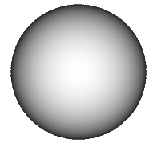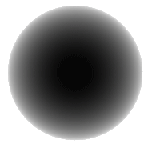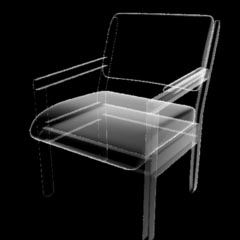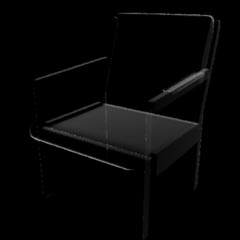### RSLEdge Effects

#### Creating Fake Lighting Effects

A surface shader generally calculates the apparent color of a surface (`Ci`) by combining the true color (`Cs`), apparent opacity (`Oi`) and surface illumination. This tutorial shows how it is possible to create the illusion of soft rim lighting without the use of any light sources.

#### Basic Code

To keep the code developed in this tutorial as simple as possible the shader shown below does not calculate the true values of the ambient, diffuse and specular lighting components. Instead, it applies a fake (diffuse) rim lighting effect based on the angle between the viewing vector and the surface normal.

We can begin with the minimalist source code shown in listing 1.The rib file used as the basis of the experiments in this tutorial is shown in listing 2.

Listing 1

 ```surface rim(float Kd = 1) { /* STEP 1 - make a copy of the surface normal one unit in length */ normal n = normalize(N); normal nf = faceforward(n, I, n); /* STEP 2 - set the apparent surface opacity */ Oi = Os; /* STEP 3 - set the fake diffuse "lighting" */ color diffusecolor = 1; /* STEP 4 - calculate the apparent surface color */ Ci = Oi * Cs * diffusecolor; }```

Listing 2

 ```Display "rim_tester" "framebuffer" "rgb" Format 240 240 1 Projection "perspective" "fov" 40 ShadingRate 1 Translate 0 0 4 Rotate -30 1 0 0 Rotate 0 0 1 0 Scale 1 1 -1 WorldBegin TransformBegin Surface "rim" "float Kd" 1 Sphere 1 -1 1 360 TransformEnd WorldEnd ```

#### Using the Viewing Vector and Surface Normal

The following illustration shows how the angle between the surface normal and the line-of-sight of the camera ie. the viewing vector, changes from 0.0 degrees at the center of the object (location A) to 90.0 degrees at the rim (location B).The apparent brightness of a surface can be based on the way this angle (shown in blue) changes across an object. A convenient method of calculating this angle is via the vector multiplication known as the dot product (also called the scalar or inner product) ie.

Code Snippet 1

 ```vector i = normalize(-I); diffusecolor = nf.i;```Figure 2

 The snippet of code shown above uses normalized copies of the surface normal (`nf`) and the (reversed) viewing vector (`i`). The dot product yields the cosine of the angle between the two vectors. The shading effect is shown in figure 2. Inverting the values by subtracting the dot product from 1.0 gives the shading effect shown in figure 3.

Code Snippet 2

 ```vector i = normalize(-I); diffusecolor = 1 - nf.i;```Figure 3

 The important point to note is that because the normal used in these calculations has been forced to face the camera, and hence we are only dealing with angles between 0.0 and 90 degrees, the cosines of this range of angles, calculated by the dot product, is in the rangle 0.0 to 1.0. In other words we are applying a fake lighting effect.

#### Controlling the Width of the Rim Effect

Naturally, the shader should allow an artist to control the width of the rim effect. By providing an instance variable, say, rim_width we can use the smoothstep() function to modify the range of values across a surface. For example,

Code Snippet 3

 ```float dot = 1 - nf.i; diffusecolor = smoothstep(1.0 - rim_width, 1.0, dot);```

An interesting effect, figure 4, can also be obtained by using the dot product to vary the opacity of a surface rather than its color (snippet 4).

Code Snippet 4

 ```float dot = 1 - nf.i; Oi = 1 - smoothstep(1.0 - rim_width, 1.0, dot);```

Listing 3 provides the full code of a shader that applied the edge effect seen in figure 4.Figure 4

Listing 3

 ```surface rim(float Kd = 1, rim_width = 0.2) { /* STEP 1 - make a copy of the surface normal one unit in length */ normal n = normalize(N); normal nf = faceforward(n, I, n); /* STEP 2 - set the apparent surface opacity */ vector i = normalize(-I); float dot = 1 - i.nf; Oi = smoothstep(1 - rim_width, 1.0, dot); /* STEP 3 - set diffusecolor to white */ color diffusecolor = 1; /* STEP 4 - calculate the apparent surface color */ Ci = Oi * Cs * diffusecolor; }```

#### Fake Lighting Direction

The chair in figure 5 has been rendered using the shader shown in listing 4. Notice that step 2 consists of two parts. In step 2.1 a uniform value of `Oi` is obtained. In step 2.2, `Oi` is scaled by the dot product of the surface normal and instance variable direction - this provides an illusion of a (fake) lighting direction.

Listing 4

 ```surface rim(float Kd = 1, rim_width = 0.2; vector direction = vector(0,-1,0)) { /* STEP 1 - make a copy of the surface normal one unit in length */ normal n = normalize(N); normal nf = faceforward(n, I); /* STEP 2.1 - set the apparent surface opacity */ vector i = normalize(-I); float dot = 1 - i.nf; Oi = smoothstep(1 - rim_width, 1.0, dot); /* STEP 2.2 - modify the opacity by "direction" */ dot = normalize(direction).n; Oi *= smoothstep(1 - rim_width, 1.0, dot); /* STEP 3 - set diffusecolor to white */ color diffusecolor = 1; /* STEP 4 - calculate the apparent surface color */ Ci = Oi * Cs * diffusecolor; }```Figure 5